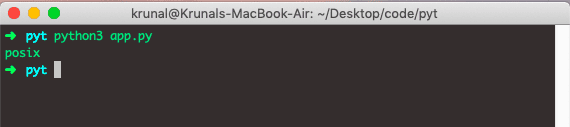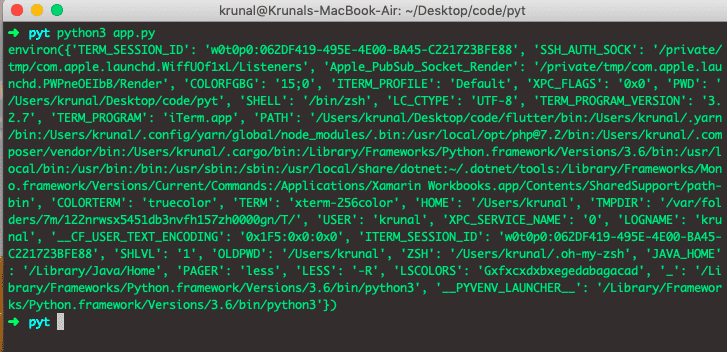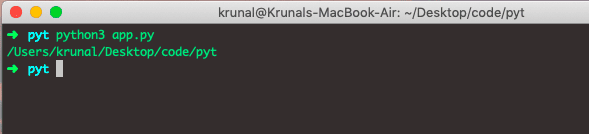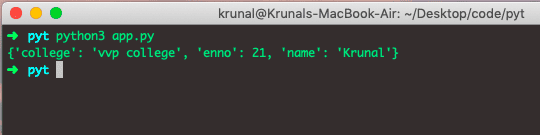# Python OS Module

Python OS module provides a way of using operating system-dependent functionality. The OS module’s functions allow you to interface with the underlying operating system that Python is running on: Windows, macOS, or Linux.

``import os``

## os.name()

The os.name() function gives the name of the OS module it imports. This differs based on the underlying Operating System.

### Example

``````import os

print(os.name)
``````

Output## os.environ()

The environ is not a function but a process parameter through which we can access the environment variables of the system.

### Example

``````import os

print(os.environ)``````

Output## os.getcwd()

The getcwd function of the OS module will give us the current directory of the project.

### Example

``````import os

print(os.getcwd())``````

OutputIf you want to make a new directory, type the following code.

## os.execvp()

The execvp() function is one of the ways to run other commands on the system. Let’s see the following example.

### Example

``````student = {
'name': 'Krunal',
'enno': 21,
'college': 'vvp college'
}

print(student)``````

Now, write the following code inside the app.py file.

``````import os

program = 'python'
arguments = ['mod.py']
print(os.execvp(program, (program,) + tuple(arguments)))``````

OutputThat’s it.

This site uses Akismet to reduce spam. Learn how your comment data is processed.